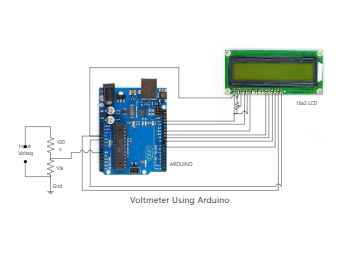Template Community / Voltmeter Using Arduino Diagram

# Voltmeter Using Arduino Diagram

Share
Duplicate17
0
Report
Publish time：09-30-2021
You can easily measure the voltage using a voltmeter, But you can also measure the DC voltage by using Arduino and a 16x2 liquid crystal display. Simply you have to set the connections of display and Arduino as shown in the diagram and make a voltage divider which is sensed by an Arduino analog pin. But You might be thinking that what is Arduino? Don't worry Arduino is just a simple microcontroller that starts working when any command and programming is installed in it. Simply you have to program the Arduino for desired projects. How does Arduino Voltmeter Works? The digital pins of Arduino are connected to the liquid crystal display to give the measurements reading which is calculated by the microcontroller and as a set in the coding. We have to set a voltage divider among the analog pin of audio to know which senses the input voltage and gives the correct reading at the output. 3) 16x2 LCDCaptain O CaptainPost
Recommended Templates